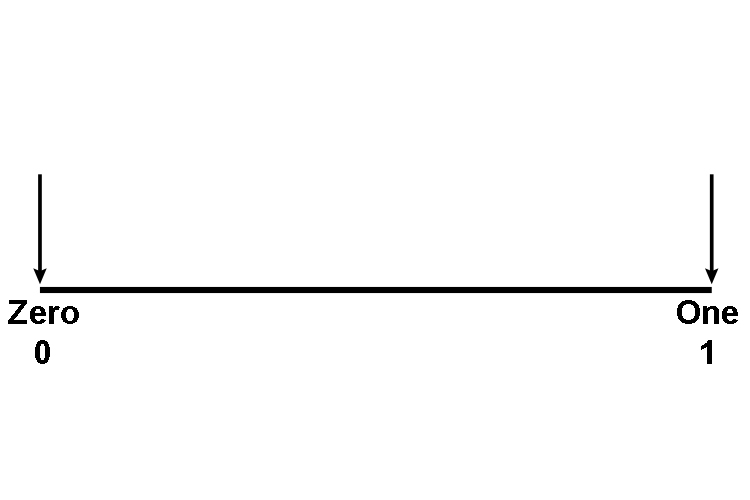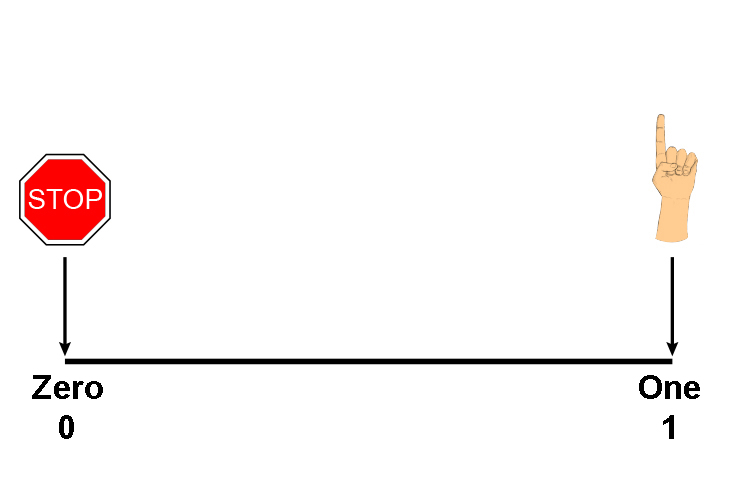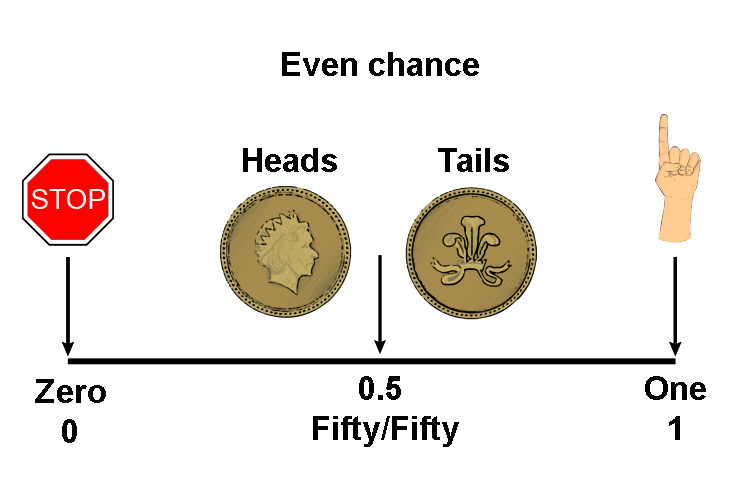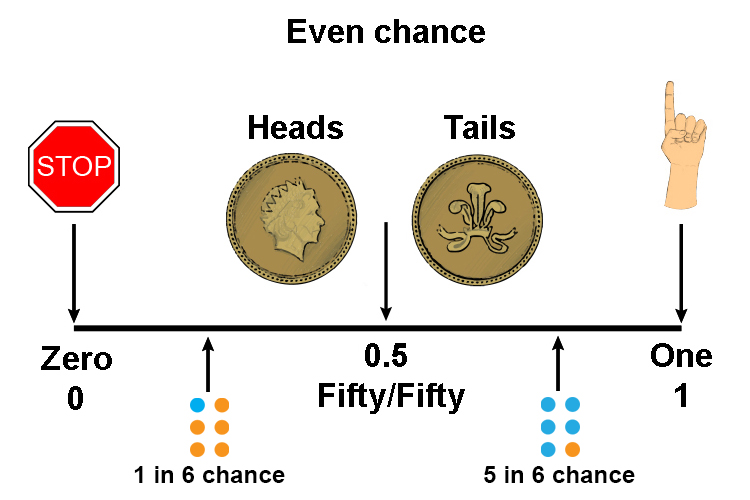# Probability line

## Probability can be shown on a line

Remember the highest probability = 1

Remember the lowest probability = 0

So draw a line with these at one end:Figure out what the middle would give you (1/2=0.5)